PRINTABLE FOR KIDS

XII (12) HSC

XI (11) FYJC
X (10) SSC

# Study Of Sound

##### Class 9th Science & Technology MHB Solution
Exercises
1. Sound does not travel through ………… Fill in the blanks and explain.…
2. The velocity of sound in steel is …............ than the velocity of sand in water. Fill…
3. The incidence of ……… in daily life shows that the velocity of sound is less than the…
4. To discover a sunken ship or objects deep inside the sea, .......................…
5. Explain giving scientific reasons. A. The roof of a movie theatre and a conference hall is…
6. Answer the following questions in your own words. A. What is an echo? What factors are…
7. Where and why are sound absorbing materials used?
8. The speed of sound in air at 0^0 C is 332 m/s. If it increases at the rate of 0.6 m/s per…
9. Nita heard the sound of lightning after 4 seconds of seeing it. What was the distance of…
10. Sunil is standing between two walls. The wall closest to him is at a distance of 360 m. If…
11. Hydrogen gas is filled in two identical bottles, A and B, at the same temperature. The…
12. Helium gas is filled in two identical bottles A and B. The mass of the gas in the two…

###### Exercises
Question 1.

Fill in the blanks and explain.

Sound does not travel through …………

Vacuum

Explanation: Sound is a mechanical wave. As all mechanical waves require a material medium to travel, sound can also travel only in material media. As vacuum is not a material medium, sound cannot travel in vacuum.

Question 2.

Fill in the blanks and explain.

The velocity of sound in steel is …............ than the velocity of sand in water.

greater

Explanation: Velocity of sound in solids>liquids>gases. Therefore, velocity of sound in steel is greater than velocity of sound in water.

Question 3.

Fill in the blanks and explain.

The incidence of ……… in daily life shows that the velocity of sound is less than the velocity of light

lightning during thunders

Explanation: During thundering, we observe lighting first and the sound is heard later. This proves that velocity of light is greater than velocity of sound.

Question 4.

Fill in the blanks and explain.

To discover a sunken ship or objects deep inside the sea, ....................... technology is used.

SONAR(Sound Navigation and Ranging)

Explanation: SONAR is used to determine the direction, distance and speed of an underwater object with the help of ultra sonic sound waves.

Question 5.

Explain giving scientific reasons.

A. The roof of a movie theatre and a conference hall is curved.

B. The intensity of reverberation is higher in a closed and empty house.

C. We cannot hear the echo produced in a classroom

A. Reason: Sound waves get reflected from the roof multiple times. This causes a single sound to be heard continuously. This is called reverberation. The time between successive reflections of a particular sound wave reaching us becomes smaller and the reflected sounds get mixed up and produce a continuous sound of increased loudness which cannot be deciphered clearly. To avoid this phenomenon, the roof of a movie theatre and conference hall is curved.

B. Reason: In a closed room, there are many objects or obstructions acting as sound absorbers. So the amount of sound getting reflected is very less. But in case of an empty room there are no obstructions. So, sound gets reflected multiple times leading to greater intensity of reverberation.

C. Reason: The dimensions of a classroom usually do not exceed 17.2m. If the dimensions are greater than 17.2m , echo is produced. So echo is not produced in a classroom.

Question 6.

Answer the following questions in your own words.

A. What is an echo? What factors are important to get a distinct echo?

B. Study the construction of the Golghumat at Vijapur and discuss the reasons for the multiple echoes produced there.

C. What should be the dimensions and the shape of classrooms so that no echo can be produced there?

A. Echo: An echo is the repetition of original sound because of reflection by a surface.

Factors important to get a distinct echo:

The velocity of sound at 220C temperature is 344 m/s. Our brain retains a sound for 0.1 sec. So to hear a distinct echo, the time taken by the starting sound to get reflected should be greater than 0.1 sec. So the distance between the listener and the reflecting surface at this temperature should be

d = v × t

= 344 m/s × 0.1 s

= 34.4 m.

This is the distance required by the starting sound to reach the reflecting surface, get reflected and reach the listener. So, the distance between the listener and reflecting surface should be atleast half of the above calculated distance i.e. 17.2km.

B. In GolGumbaz and Vijaypur the reason for multiple echoes produced is due to multiple reflections of sound by the tomb. The sound produced gets reflected several times throughout the tomb which is responsible for echos.

C. A classroom should be rectangular in shape and its dimensions should be less than 17.2m for an echo to be not produced at normal room temperature.

Sound absorbing material like should be also used for soundproofing the room.

Question 7.

Where and why are sound absorbing materials used?

Sound absorbing materials are used in buildings and offices to prevent transmission or reflection of sound. They prevent leakage of sound to other rooms. Sound absorbing materials also prevent echoes.

Question 8.

Solve the following examples.

The speed of sound in air at 00C is 332 m/s. If it increases at the rate of 0.6 m/s per degree, what will be the temperature when the velocity has increased to 344 m/s?

Given:

Speed of sound in air at 00C = 332m/s

Speed increases at a rate of 0.6m/s per degree

Temperature at 344 m/s = T0c

Difference in velocities = 344 m/s - 332 m/s

= 12 m/s

Increase in temperature corresponding to the increase in velocity =

12/0.6 = 200C

Therefore temperature at velocity 344m/s = 00C + 200C = 200C

Question 9.

Solve the following examples.

Nita heard the sound of lightning after 4 seconds of seeing it. What was the distance of the lightning from her?

Given:

Velocity of sound in air (v) = 340 m/s2

Time taken for the sound to reach the receiver(t) = 4 sec

Distance of the source from receiver (d) = v × t

= 340 × 4

= 1360 m/s

Question 10.

Solve the following examples.

Sunil is standing between two walls. The wall closest to him is at a distance of 360 m. If he shouts, he hears the first echo after 4 s and another after another 2 seconds.

1. What is the velocity of sound in air?

2. What is the distance between the two walls?

Given:

Distance of nearest wall from the man (d) = 360m

Time after which the man hears the first echo (t1) = 4s

Time after which the man hears the second echo (t2) = 4s+2s = 6s

1.Distance travelled by sound when man hears the first echo = 2d1 = 720m

Velocity of sound = Distance travelled by sound/Time taken

= 720/4

= 180 m/s

2. Let distance between two walls = x m

Distance between second wall and the man(d2) = (x-360)m

Time taken for the second echo to reach the man (t2) = 6s

Distance travelled by the sound = 2d2

Velocity of sound = Distance travelled by sound/Time taken

180 = (2(x-360))/6

x = 900 m

Therefore, distance between the two walls (x) = 900m

Question 11.

Solve the following examples.

Hydrogen gas is filled in two identical bottles, A and B, at the same temperature. The mass of hydrogen in the two bottles is 12gm and 48 gm respectively. In which bottle will sound travel faster? How many times as fast as the other?

Given:

Mass of hydrogen in bottle A (MA) = 12gm

Mass of hydrogen in bottle B (MB) = 48gm

We know that mass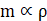So ρAB = MA/MB

We know that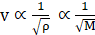Therefore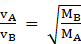So, vA = 2vB

So velocity in bottle B is two times the velocity in bottle A.

Question 12.

Solve the following examples.

Helium gas is filled in two identical bottles A and B. The mass of the gas in the two bottles is 10 gm and 40 gm respectively. If the speed of sound is the same in both bottles, what conclusions will you draw?

Given:

Mass of helium in bottle A = 10 gm

Mass of helium in bottle B = 40 gm

Velocity of helium in bottle A = Velocity of helium in bottle B

= > vA = vB = v

We know that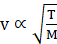T = Temperature

So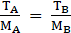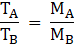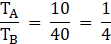Temperature in bottle A is less than temperature in bottle B.

## PDF FILE TO YOUR EMAIL IMMEDIATELY PURCHASE NOTES & PAPER SOLUTION. @ Rs. 50/- each (GST extra)

SUBJECTS

HINDI ENTIRE PAPER SOLUTION

MARATHI PAPER SOLUTION
SSC MATHS I PAPER SOLUTION
SSC MATHS II PAPER SOLUTION
SSC SCIENCE I PAPER SOLUTION
SSC SCIENCE II PAPER SOLUTION
SSC ENGLISH PAPER SOLUTION
SSC & HSC ENGLISH WRITING SKILL
HSC ACCOUNTS NOTES
HSC OCM NOTES
HSC ECONOMICS NOTES
HSC SECRETARIAL PRACTICE NOTES

2019 Board Paper Solution

HSC ENGLISH SET A 2019 21st February, 2019

HSC ENGLISH SET B 2019 21st February, 2019

HSC ENGLISH SET C 2019 21st February, 2019

HSC ENGLISH SET D 2019 21st February, 2019

SECRETARIAL PRACTICE (S.P) 2019 25th February, 2019

HSC XII PHYSICS 2019 25th February, 2019

CHEMISTRY XII HSC SOLUTION 27th, February, 2019

OCM PAPER SOLUTION 2019 27th, February, 2019

HSC MATHS PAPER SOLUTION COMMERCE, 2nd March, 2019

HSC MATHS PAPER SOLUTION SCIENCE 2nd, March, 2019

SSC ENGLISH STD 10 5TH MARCH, 2019.

HSC XII ACCOUNTS 2019 6th March, 2019

HSC XII BIOLOGY 2019 6TH March, 2019

HSC XII ECONOMICS 9Th March 2019

SSC Maths I March 2019 Solution 10th Standard11th, March, 2019

SSC MATHS II MARCH 2019 SOLUTION 10TH STD.13th March, 2019

SSC SCIENCE I MARCH 2019 SOLUTION 10TH STD. 15th March, 2019.

SSC SCIENCE II MARCH 2019 SOLUTION 10TH STD. 18th March, 2019.

SSC SOCIAL SCIENCE I MARCH 2019 SOLUTION20th March, 2019

SSC SOCIAL SCIENCE II MARCH 2019 SOLUTION, 22nd March, 2019

XII CBSE - BOARD - MARCH - 2019 ENGLISH - QP + SOLUTIONS, 2nd March, 2019

HSC Maharashtra Board Papers 2020

(Std 12th English Medium)

HSC ECONOMICS MARCH 2020

HSC OCM MARCH 2020

HSC ACCOUNTS MARCH 2020

HSC S.P. MARCH 2020

HSC ENGLISH MARCH 2020

HSC HINDI MARCH 2020

HSC MARATHI MARCH 2020

HSC MATHS MARCH 2020

SSC Maharashtra Board Papers 2020

(Std 10th English Medium)

English MARCH 2020

HindI MARCH 2020

Hindi (Composite) MARCH 2020

Marathi MARCH 2020

Mathematics (Paper 1) MARCH 2020

Mathematics (Paper 2) MARCH 2020

Sanskrit MARCH 2020

Important-formula

THANKS